# Ordering fractions worksheet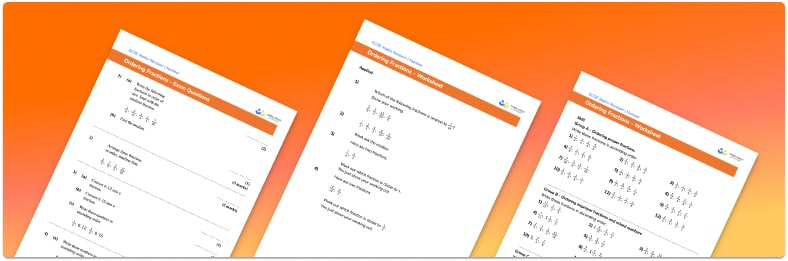• Section 1 of the ordering fractions printable worksheet contains 20+ skills-based ordering fractions questions, in 3 groups to support differentiation
• Section 2 contains 3 applied ordering fractions questions with a mix of worded problems and deeper problem solving questions
• Section 3 contains 3 foundation and higher level GCSE exam style ordering fractions questions
• Answer keys and a mark scheme for all ordering fractions questions are provided
• Questions follow variation theory with plenty of opportunities for students to work independently at their own level
• All questions created by fully qualified expert secondary maths teachers
• Suitable for GCSE maths revision for AQA, OCR and Edexcel exam boards

• This field is for validation purposes and should be left unchanged.

You can unsubscribe at any time (each email we send will contain an easy way to unsubscribe). To find out more about how we use your data, see our privacy policy.

### Ordering fractions at a glance

Sometimes we want to order or compare fractions. If fractions have the same denominator, this is easy as we can simply compare the numerators. For example, we know 7 divided by 9 is bigger than 5 divided by 9. However, if the fractions have different denominators, comparing the fractions is not so easy.

In order to accurately compare fractions, we need to write them with a common denominator. To do this, we find the lowest common multiple of the denominators of the fractions and find equivalent fractions to each, all with the lowest common multiple as denominator. For example, we can compare 3 fifths and 4 sevenths by finding equivalent fractions with a denominator of 35. 3 fifths is equal to 21 thirty-fifths and 4 sevenths is equal to 20 thirty-fifths. We can now easily see that 3 fifths is bigger than 4 sevenths.

When ordering a set of fractions, once we have written all of the fractions with like denominators, we can easily arrange the fractions into ascending or descending order.

A visual model using fraction circles can help students to understand equivalent fractions.

Looking forward, students can then progress to additional comparing fractions worksheets and other number worksheets, for example a multiplying and dividing decimals worksheet or an order of operations worksheet.For more teaching and learning support on Number our GCSE maths lessons provide step by step support for all GCSE maths concepts.

## Related worksheets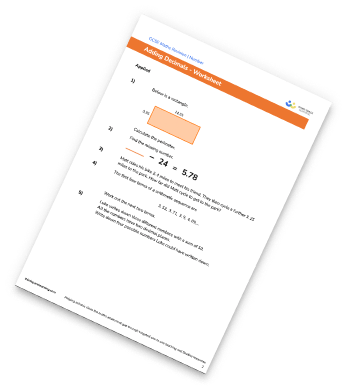Subtracting decimals worksheet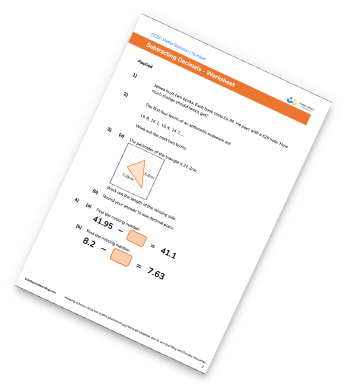Rational And Irrational Numbers Worksheet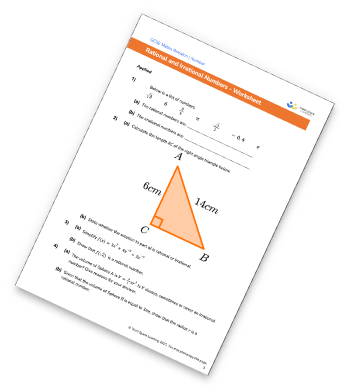Surds Worksheet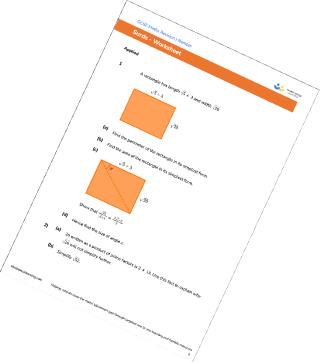## Do you have KS4 students who need more focused attention to succeed at GCSE?There will be students in your class who require individual attention to help them succeed in their maths GCSEs. In a class of 30, it’s not always easy to provide.

Help your students feel confident with exam-style questions and the strategies they’ll need to answer them correctly with our dedicated GCSE maths revision programme.

Lessons are selected to provide support where each student needs it most, and specially-trained GCSE maths tutors adapt the pitch and pace of each lesson. This ensures a personalised revision programme that raises grades and boosts confidence.

Find out more